# RC Circuit

 Impedance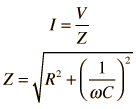Calculate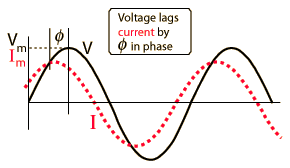Examine Capacitor Resistor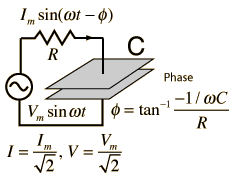Contribution tocomplex impedance Phasor diagram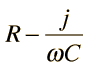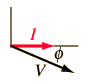You know that the voltage in a capacitive lags the current because the current must flow to build up the charge, and the voltage across the capacitor is proportional to that charge which is built up on the capacitor plates.

Index

Capacitance concepts

Inductance concepts

 HyperPhysics***** Electricity and Magnetism R Nave
Go Back

# RC Impedance

The frequency dependent impedance of an RC series circuit.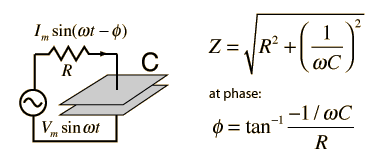For C = x10^ F = μF = pF
 at angular frequency ω = x10^ rad/s,
 frequency = x10^ Hz = kHz = MHz
 and resistance R = x10^ ohms = kohms = Megohms,

the impedance is

 Z = x10^ ohms = kohms = Megohms

 at phase φ = degrees.
Default values will be entered for unspecified parameters, but all component values can be changed. Click outside the box after entering data to initiate the calculation.
 AC behavior of RC circuit
Index

Capacitance concepts

Inductance concepts

 HyperPhysics***** Electricity and Magnetism R Nave
Go Back